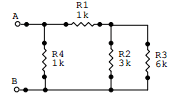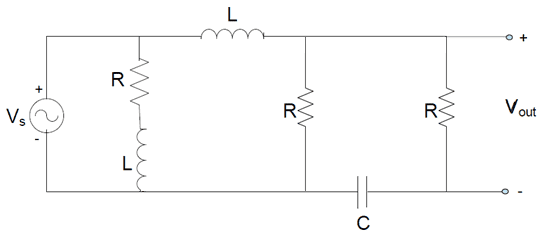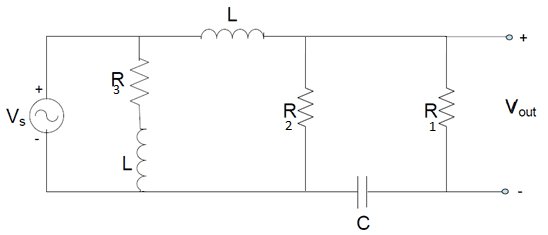# What's the difference between series an parallel components in the pictures

hi;
When I study - if we want to connect impedance in the case of: - we connect the R4 and (R1+(R2||R3)) as parallelsbut when we want the get impedance of C and R of the right: - we connect that as series: Z(of C)+R .. why the different? please help!phinds
Gold Member
Because inductance and resistance are different things and you cannot combine them as exactly the same the way you can when everything is just resistances.

berkeman
Mentor
hi;
When I study - if we want to connect impedance in the case of: - we connect the R4 and (R1+(R2||R3)) as parallels
View attachment 113258

but when we want the get impedance of C and R of the right: - we connect that as series: Z(of C)+R .. why the different? please help!
View attachment 113259
In the 2nd circuit, it's not clear what you are trying to solve for. In general, that circuit will not combine down to a simple component or two. Could you label the different components with different numbers (so each resistor has a different number, etc.), and ask what you would like to be able to solve for in the circuit?

In the 2nd circuit, it's not clear what you are trying to solve for. In general, that circuit will not combine down to a simple component or two. Could you label the different components with different numbers (so each resistor has a different number, etc.), and ask what you would like to be able to solve for in the circuit?I want to find a simple arranged of total impedance on the
different circuit 1 (all R) and 2 (with the C and L).

in case 1 told me that R4 and (R1+(R2||R3)) as parallels. and in case 2 impedance R1 and impedance C is series. but I think about it if I will connect something to the Terminals the connection of R1 and C is like a Δ transform with R2 no?

(may that matter what the purpose of tow cases - case 1 what the total R , and case 2 what the transfer function. If that matter i want to know why)

berkeman
Mentor
View attachment 113261
I want to find a simple arranged of total impedance on the
different circuit 1 (all R) and 2 (with the C and L).

in case 1 told me that R4 and (R1+(R2||R3)) as parallels. and in case 2 impedance R1 and impedance C is series. but I think about it if I will connect something to the Terminals the connection of R1 and C is like a Δ transform with R2 no?

(may that matter what the purpose of tow cases - case 1 what the total R , and case 2 what the transfer function. If that matter i want to know why)
The impedance for circuit 2 looking into which 2 nodes? It makes a difference...phinds